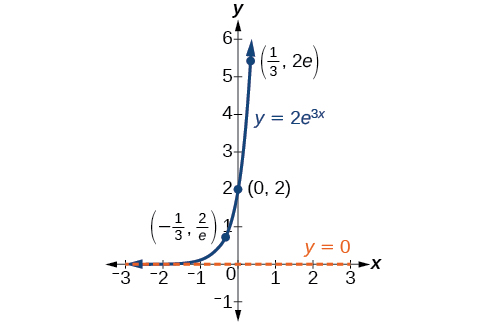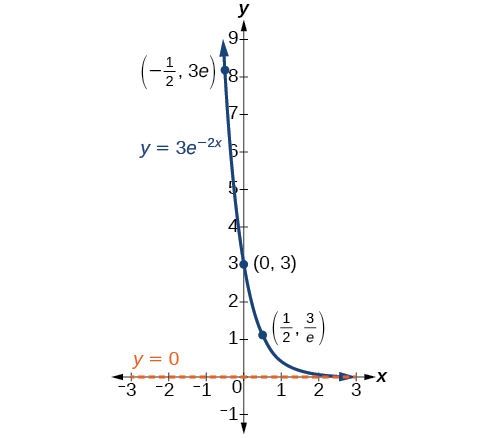# 6.7 Exponential and logarithmic models

 Page 1 / 16
In this section, you will:
• Model exponential growth and decay.
• Use Newton’s Law of Cooling.
• Use logistic-growth models.
• Choose an appropriate model for data.
• Express an exponential model in base $\text{\hspace{0.17em}}e$ .A nuclear research reactor inside the Neely Nuclear Research Center on the Georgia Institute of Technology campus (credit: Georgia Tech Research Institute)

We have already explored some basic applications of exponential and logarithmic functions. In this section, we explore some important applications in more depth, including radioactive isotopes and Newton’s Law of Cooling.

## Modeling exponential growth and decay

In real-world applications, we need to model the behavior of a function. In mathematical modeling, we choose a familiar general function with properties that suggest that it will model the real-world phenomenon we wish to analyze. In the case of rapid growth, we may choose the exponential growth function:

$y={A}_{0}{e}^{kt}$

where ${A}_{0}$ is equal to the value at time zero, $e$ is Euler’s constant, and $k$ is a positive constant that determines the rate (percentage) of growth. We may use the exponential growth    function in applications involving doubling time , the time it takes for a quantity to double. Such phenomena as wildlife populations, financial investments, biological samples, and natural resources may exhibit growth based on a doubling time. In some applications, however, as we will see when we discuss the logistic equation, the logistic model sometimes fits the data better than the exponential model.

On the other hand, if a quantity is falling rapidly toward zero, without ever reaching zero, then we should probably choose the exponential decay model. Again, we have the form $y={A}_{0}{e}^{kt}$ where ${A}_{0}$ is the starting value, and $e$ is Euler’s constant. Now $k$ is a negative constant that determines the rate of decay. We may use the exponential decay model when we are calculating half-life    , or the time it takes for a substance to exponentially decay to half of its original quantity. We use half-life in applications involving radioactive isotopes.

In our choice of a function to serve as a mathematical model, we often use data points gathered by careful observation and measurement to construct points on a graph and hope we can recognize the shape of the graph. Exponential growth and decay graphs have a distinctive shape, as we can see in [link] and [link] . It is important to remember that, although parts of each of the two graphs seem to lie on the x -axis, they are really a tiny distance above the x -axis.A graph showing exponential growth. The equation is y = 2 e 3 x .A graph showing exponential decay. The equation is y = 3 e − 2 x .

Exponential growth and decay often involve very large or very small numbers. To describe these numbers, we often use orders of magnitude. The order of magnitude    is the power of ten, when the number is expressed in scientific notation, with one digit to the left of the decimal. For example, the distance to the nearest star, Proxima Centauri , measured in kilometers, is 40,113,497,200,000 kilometers. Expressed in scientific notation, this is $4.01134972\text{\hspace{0.17em}}×\text{\hspace{0.17em}}{10}^{13}.$ So, we could describe this number as having order of magnitude ${10}^{13}.$

find the value of 2x=32
divide by 2 on each side of the equal sign to solve for x
corri
X=16
Michael
Want to review on complex number 1.What are complex number 2.How to solve complex number problems.
Beyan
use the y -intercept and slope to sketch the graph of the equation y=6x
how do we prove the quadratic formular
hello, if you have a question about Algebra 2. I may be able to help. I am an Algebra 2 Teacher
thank you help me with how to prove the quadratic equation
Seidu
may God blessed u for that. Please I want u to help me in sets.
Opoku
what is math number
4
Trista
x-2y+3z=-3 2x-y+z=7 -x+3y-z=6
Need help solving this problem (2/7)^-2
x+2y-z=7
Sidiki
what is the coefficient of -4×
-1
Shedrak
the operation * is x * y =x + y/ 1+(x × y) show if the operation is commutative if x × y is not equal to -1
An investment account was opened with an initial deposit of \$9,600 and earns 7.4% interest, compounded continuously. How much will the account be worth after 15 years?
lim x to infinity e^1-e^-1/log(1+x)
given eccentricity and a point find the equiation
12, 17, 22.... 25th term
12, 17, 22.... 25th term
Akash
College algebra is really hard?
Absolutely, for me. My problems with math started in First grade...involving a nun Sister Anastasia, bad vision, talking & getting expelled from Catholic school. When it comes to math I just can't focus and all I can hear is our family silverware banging and clanging on the pink Formica table.
Carole
I'm 13 and I understand it great
AJ
I am 1 year old but I can do it! 1+1=2 proof very hard for me though.
Atone
Not really they are just easy concepts which can be understood if you have great basics. I am 14 I understood them easily.
Vedant
hi vedant can u help me with some assignments
Solomon
find the 15th term of the geometric sequince whose first is 18 and last term of 387
I know this work
salmaByBy RhodesBy RhodesByByByByBy